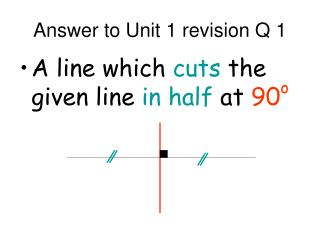# Answer to Unit 1 revision Q 1 - PowerPoint PPT PresentationDownload PresentationAnswer to Unit 1 revision Q 1

Answer to Unit 1 revision Q 1
Download Presentation## Answer to Unit 1 revision Q 1

- - - - - - - - - - - - - - - - - - - - - - - - - - - E N D - - - - - - - - - - - - - - - - - - - - - - - - - - -
##### Presentation Transcript

1. Answer to Unit 1 revision Q 1 • A line which cuts the given line in half at 90o

2. A C B M Answer to Unit 1 revision Q 2 • (i) find the mid point of BC. Call it M (ii) find the gradient of AM (iii) use y-b = m(x-a)

3. Answer to Unit 1 revision Q 4 • Simultaneous equations

4. y x Answer to Unit 1 revision Q 3 • undefined

5. y (a,b) x Answer to Unit 1 revision Q 6 • y – b = m(x – a)

6. y (a,b) m 1 x Answer to Unit 1 revision Q 5 • The gradient of the line and a point on the line

7. y x Answer to Unit 1 revision Q 8 • 0

8. y Θ x Answer to Unit 1 revision Q 7 • m = tan Θ

9. Answer to Unit 1 revision Q 10 • Divide by 180 and multiply by π

10. Answer to Unit 1 revision Q 9 • A line from a vertex, perpendicular to the opposite side

11. Answer to Unit 1 revision Q 12 • (i) rearrange to sinx = (ii) decide on 2 quadrants (iii) ignore any –ve and press INV sin to get angle (iv) work out two answers

12. Answer to Unit 1 revision Q 11 • x = logay

13. x b a Answer to Unit 1 revision Q 14 • (i) draw triangle (ii) use Pythagoras to fill in missing side (iii) read values off triangle using SOHCAHTOA

14. Answer to Unit 1 revision Q 13 • move graph up 3

15. Answer to Unit 1 revision Q 16 • (1,0) and (a,1)

16. Answer to Unit 1 revision Q 15 • Reflect the graph in the x-axis

17. Answer to Unit 1 revision Q 18 • (i) differentiate (ii) show that f’(x) is always negative e.g. –(x+a)2 , x = a

18. Answer to Unit 1 revision Q 20 • -1 < a < 1

19. Answer to Unit 1 revision Q 19 (i) differentiate (ii) let f’(x) = 0 (iii) solve to find stationary points (iv) find y-coordinates (v) draw nature table

20. Answer to Unit 1 revision Q 22 • (i) differentiate (ii) show that f’(x) is always positive e.g. (x+a)2 , x = a

21. Answer to Unit 1 revision Q 21 • Reflect y = f ( x ) in the y axis.

22. Answer to Unit 1 revision Q 24 • cos x = ±√3 2 x =300,1500,2100or 3300

23. Answer to Unit 1 revision Q 23 • x ( x – 6 )( x + 4 ) = 0 so x = 0, x = 6 or x = 4